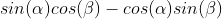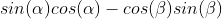Trigonometry : Sum and Difference of Sines and Cosines

Example Questions

Example Question #1 : Sum And Difference Of Sines And Cosines

What is the correct formula for the sum of two sines: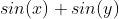?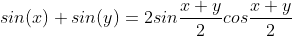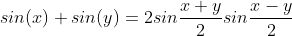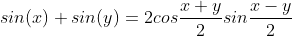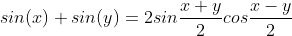Explanation:

This is a known trigonometry identity.  Whenever you are adding two sine functions, you can plug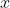and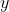into the formula to solve for this sum

Example Question #2 : Sum And Difference Of Sines And Cosines

Solve for the following given that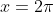.  Use the formula for the sum of two sines.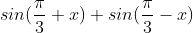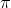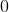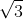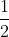Explanation:

We begin by considering our formula for the sum of two sinesWe will let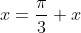and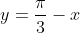and plug these values into our formula.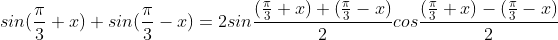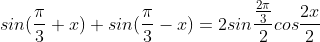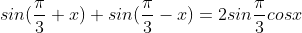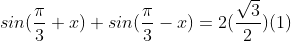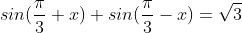Example Question #3 : Sum And Difference Of Sines And Cosines

Solve for the following using the formula for the differences of two cosines.  Do not simplify.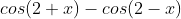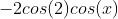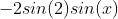Explanation:

We begin by considering the formula for the differences of two cosines.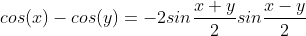We will let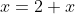and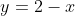.  Proceed by plugging these values into the formula.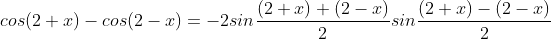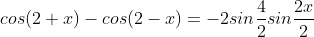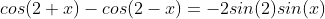Example Question #4 : Sum And Difference Of Sines And Cosines

Which of the following completes the identity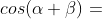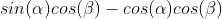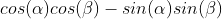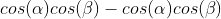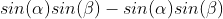Explanation:

This is a known trigonometry identity and has been proven to be true.  It is often helpful to solve for the quantity within a cosine function when there are unknowns or if the quantity needs to be simplified

Example Question #5 : Sum And Difference Of Sines And Cosines

Solve for the following using the correct identity: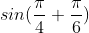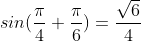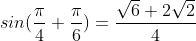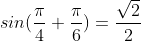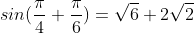Explanation:

To solve this problem we must use the identity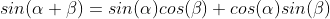.  We will let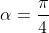and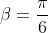.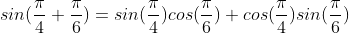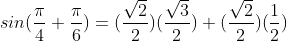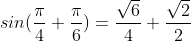Example Question #6 : Sum And Difference Of Sines And Cosines

True or False: To solve for a problem in the form of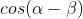, I use the identity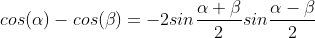.

False

True

False

Explanation:

This answer is false.is not the same as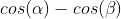.

For example, say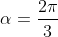and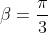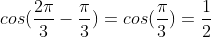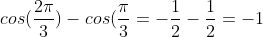And so the correct identity to use for this is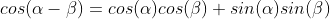Example Question #7 : Sum And Difference Of Sines And Cosines

Solve for the following using the correct identity: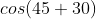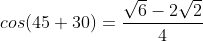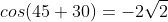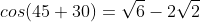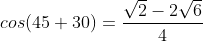Explanation:

The correct identity to use for this kind of problem is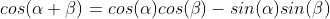We will let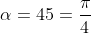and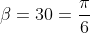.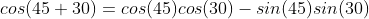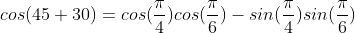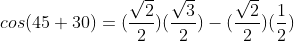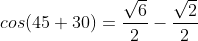Example Question #8 : Sum And Difference Of Sines And Cosines

Which of the following is the correct to complete the following identity: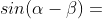___?